Starts With A Bang

# Weekend Diversion: Triangles, a Puzzle, and Beauty

#### Whether you’ve ever run across this famous “how many triangles” puzzle or not, you’re in for a treat looking at the magnificence of the solution.

“Arithmetic! Algebra! Geometry! Grandiose trinity! Luminous triangle! Whoever has not known you is without sense!” –Comte de Lautréamont

When you think about it, it’s amazing that our physical Universe makes sense at all. The fact that we can observe what’s happening, determine the laws that govern it, and predict what will happen under the same or similar circumstances is the most remarkable power that science has. If that’s what you’re doing in any aspect of your life, congratulations, you are a scientist. But that doesn’t tell us, fundamentally, what the Universe is like at its most basic level. Are we made up of point-like particles? Or are they geometric constructions? Are we ripples in the Universe itself? In a way, They Might Be Giants might be pondering exactly this in their song that I present to you this weekend,

At the root of all of this is mathematics, which is in its own way beautiful, elegant, and happens to be our foundation for making sense of the Universe. And in what appeared to be a simple puzzle, I saw an image similar to this one floating around the internet and making the rounds on Facebook.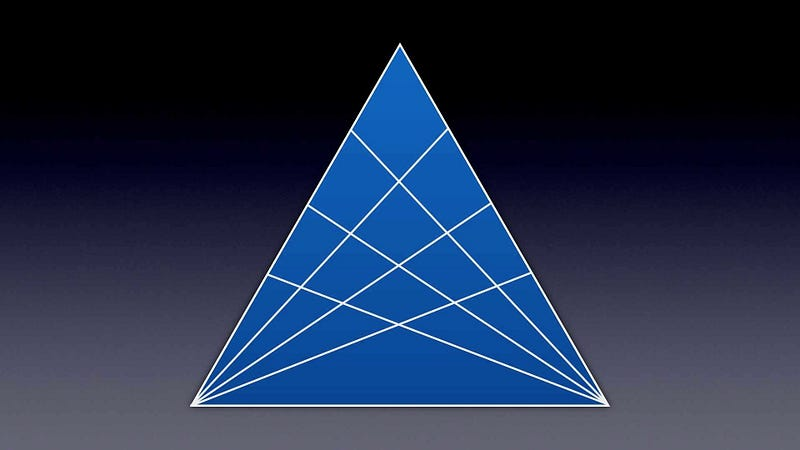How many triangles are in this image? 92.6% of Americans get this question wrong!

It’s pretty straightforward: an equilateral triangle with three extra lines coming out of two of the vertices, along with a question of “how many triangles?” can be found in this image.

Try solving it yourself, if you like, before reading on, where I’ll explain for you the correct answer, and show you a fun and beautiful math pattern that’s in there, too.

As can be expected, I saw a large number of attempts at answering this, including some fairly sophisticated erroneous ones.

It makes sense to try and construct triangles from each of the points where lines intersect, but you have to be careful not to double-or-triple count triangles. The number above is too high, as the answer isn’t seventy.

This attempted answer was particularly bothersome, because — spoiler alert — 64 is the right answer, but this diagram is totally wrong, missing some triangles that are actually there, and counting a number of triangles twice. (For example, look at the fifth row, at the red triangle in the first column, and how that’s the same as the green triangle in the sixth row, second column.)

When someone gets the right answer for the wrong reason, it’s particularly aggravating, because it takes multiple mistakes to make that happen. So I’d like to show you a foolproof method for showing you all the unique triangles in this diagram, and when we’re finished, we’ll see a pattern and get a formula to learn something fun and beautiful.

We’re going to start at the bottom of the triangle, with the two base vertices. As we move up the diagram, we’ll progressively run into points where two lines intersect, labeled above in the order we’ll run into them.

Each time we do, we’ll count all the new unique triangles by using the new, intersecting point and one (or both) of the two base vertices at the bottom of the triangle. In order to avoid double-counting, we’ll only create triangles using points below our current point, ensuring that we’ll never count the same triangle twice. You’ll also notice that some points — labeled 2 and 3, 4 and 5, 6 and 7, 9 and 10, 11 and 12, and 14 and 15 — are mirror-reflections of one another, so those sets better give us the same numbers of triangles.

Let’s go through these points, from 1 to 16, and see what we get.

For the first point we come to, there’s only one possible triangle using the points below it: there are three points in a triangle and this triangle uses all of them.

Easy enough, so it’s on to the next one(s) up.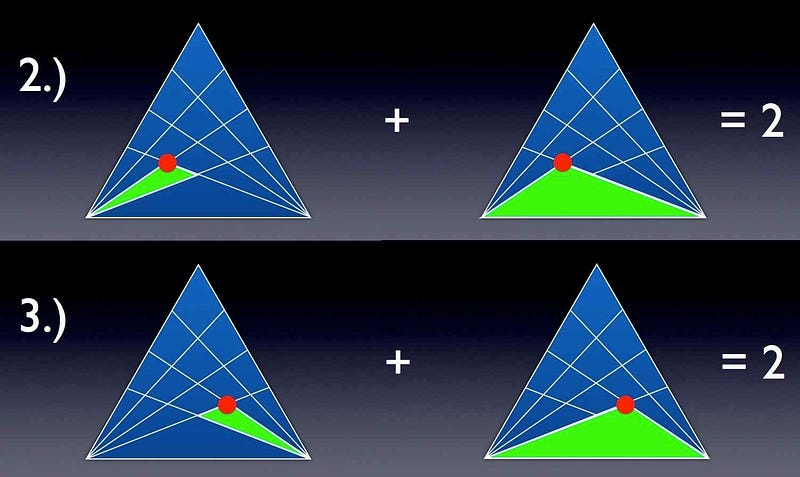Points #2 and #3 as a necessary vertex in each triangle.

As you can see, each of those new points can make two new triangles, one using both base vertices and one using our intersecting point #1, which is now an option in making a triangle. This pattern will continue as we continue to move upwards, as all lower points now become fair game.

So let’s move up to points 4 and 5.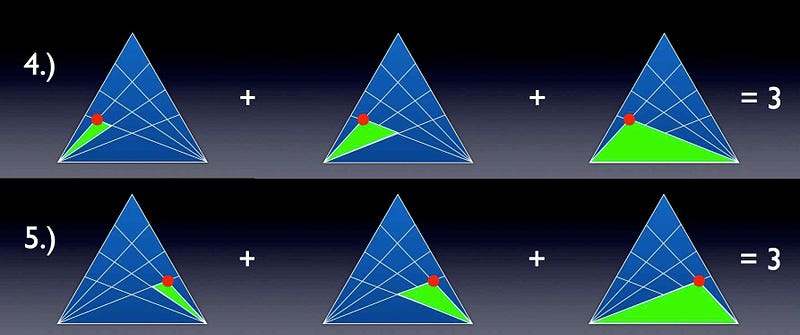Points #4 and #5 as a necessary vertex in each triangle.

There are three new triangles we can construct for each of those, as you can see. This is pretty straightforward, as are points 6 and 7, below.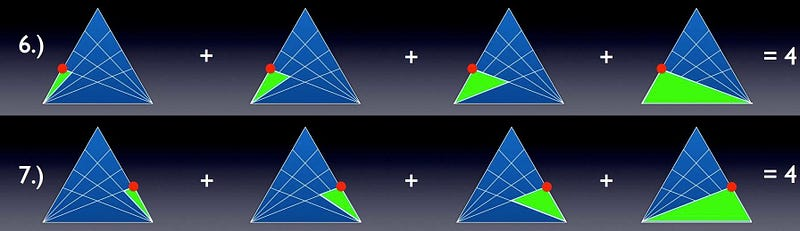Points #6 and #7 as a necessary vertex in each triangle.

Four new triangles apiece, using all of the allowable, lower points as possible vertices. So far, so good: no double-counting, and no missed triangles. And moving up one more, to intersecting point #8, finally gets a little interesting.

Why is this — point #8 — interesting as compared to the others? Because, for the first time, we can build successful, new, unique triangles that connect to either one of the base vertices, something that we’ll have to keep in mind for all of our subsequent points.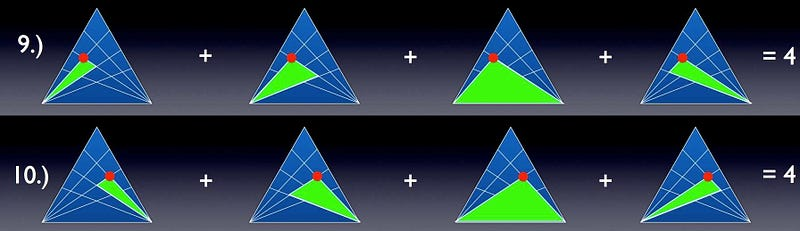Points #9 and #10 as a necessary vertex in each triangle.

Let’s move on up, and hit points 9 and 10.

Points 9 and 10 give us four new, unique triangles each, connecting to either (or both) base vertex (or vertices), as appropriate.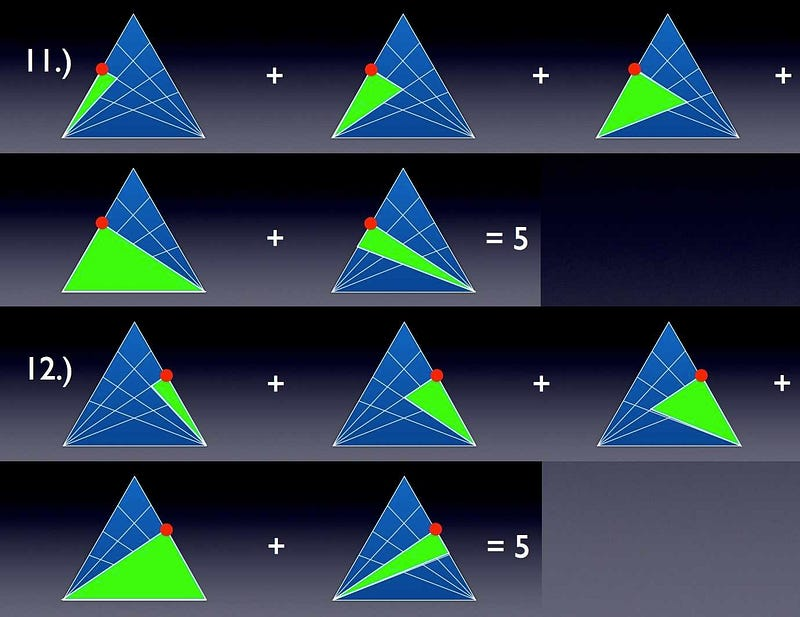Points #11 and #12 as a necessary vertex in each triangle.

And for points 11 and 12, we get five each. Feel free to check: all of these triangles, so far, are unique, and encapsulate all of them. We’ve only got four intersecting points left, so let’s take them all down!

Five more for intersecting point #13…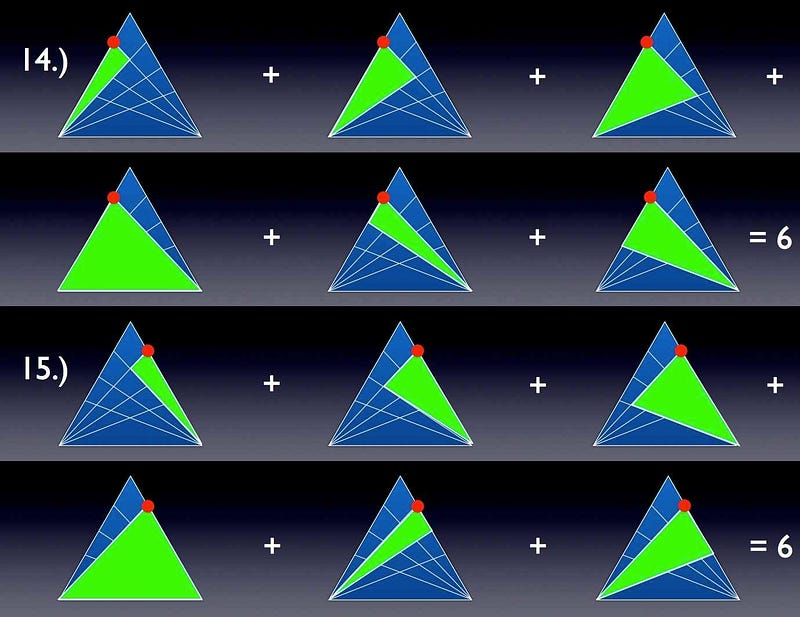Points #14 and #15 as a necessary vertex in each triangle.

Six each for points #14 and 15, and for the final, uppermost point…

Seven! All told, we can add these up, and get 1 + 2 + 2 + 3 + 3 + 4 + 4 + 3 + 4 + 4 + 5 + 5 + 5 + 6 + 6 + 7 = 64, and so there are, in fact, 64 unique triangles here.Travel the Universe with astrophysicist Ethan Siegel. Subscribers will get the newsletter every Saturday. All aboard!

Now, 64 is an interesting number: it’s a perfect square (8^2 = 64), it’s a perfect cube (4^3 = 64), and you might wonder if it’s related to the number of extra lines coming out of those two base vertices. Well, it is, but the pattern is really fantastic. Let’s show you what we get if we count the number of new triangles we were able to create — using each new point as a necessary vertex — as we moved up the triangle.

Now, that’s a beautiful pattern, and it happens to be very closely related to the number of lines — in this case, 4 — coming out of each base vertex of the triangle.

If we only had one, we’d only have the lowest line from each vertex, meaning we’d only get 1 triangle.

If we only had two, we’d have the two lowest lines from each vertex, getting a total of 8 triangles: 1 x 1 + 2 x 2 + 3 x 1 = 8.

If we only had three, we’d get the three lowest lines from each vertex, for a total of 27 triangles: 1 x 1 + 2 x 2 + 3 x 3 + 4 x 2 + 5 x 1 = 27.

And as you can see, for four, we get 64: 1 x 1 + 2 x 2 + 3 x 3 + 4 x 4 + 5 x 3 + 6 x 2 + 7 x 1 = 64.

And, as you may have noticed, 1^3 = 1, 2^3 = 8, 3^3 = 27, and 4^3 = 64, so that’s how the pattern goes! So go ahead and draw a triangle with an arbitrary number of lines coming from both vertices; you’ll not only now know the pattern, including how many triangles you can generate as each vertex as you move upwards, but you now know an awesome way to generate the perfect cubes of numbers! What a fun and beautiful little bit of math, and I hope it helps bring you not only a great weekend, but peace of mind, and closure to this epic triangle riddle!

An earlier version of this post originally appeared on the old Starts With A Bang blog at Scienceblogs.

Related
More work is needed before declaring the technique a fountain of youth.
Hinduism emphasizes the journey, whichever path that takes. And it holds us responsible for our own self-improvement.
An unexpected ancient manufacturing strategy may hold the key to designing concrete that lasts for millennia.
Quantum superposition challenges our notions of what is real.
The benefits of employee training are felt far and wide, from improvements in workers’ wellbeing to better customer interactions.

Up Next
Dan Savage: Monogamy is ridiculous and people aren’t any good at it.